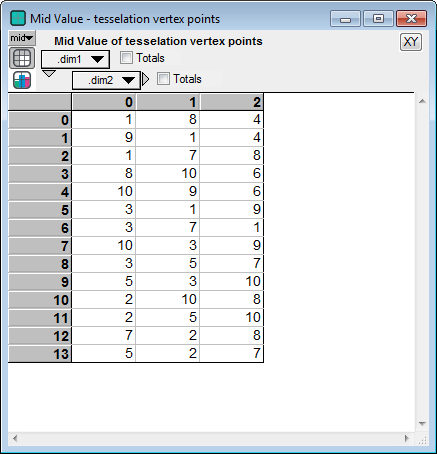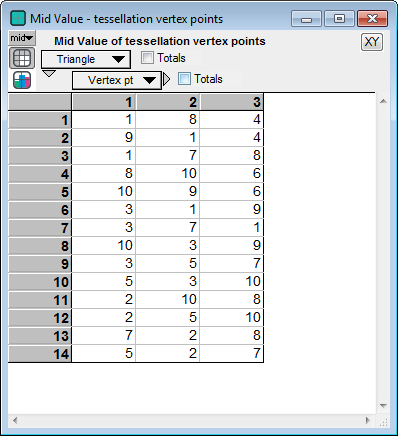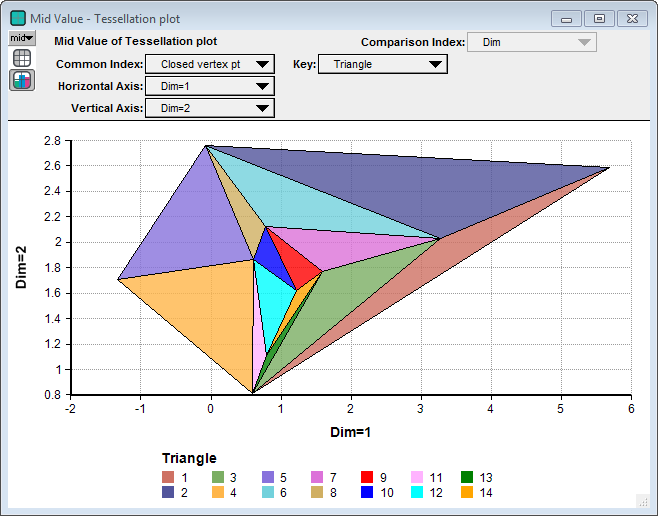# COM Integration/Calling Python code

Requires Analytica Enterprise or higher

Here's how you can call code written in the Python programming language from Analytica using the COM Integration functions.

## Calling Python code

In this example, we implement an object in Python that you can instantiate and use from an Analytica model. The object instantiates as a COM object (Component Object Model), and is available using the standard COM Integration features of Analytica Enterprise and above.

These are the basic steps to define a COM class in Python and access it from Analytica:

1. Install Python and the libraries (modules) you will need, including Analytica_Python.py.
2. Create a Python module that defines the COM class(es) <x> that you want to access from Analytica, and calls Analytica_Python.AddCOMClass(<c>) to make it (them) available as a COM object.
3. Generate a unique CLSID for each COM class at Online GUID Generator and copy it into the _reg_clsid_ attribute of the class in the Python file.
4. Register the Python COM object <c> on your computer to make it accessible to COM.
5. Access the COM object from your Analytica model using COMCreateObject(<c>)
6. If the object or its methods take array parameters, use COMArray() to convert from Analytica to Python accessible arrays in Analytica.

See below for a detailed example, which calls a function from the Python scipy.spatial library to compute a Delaunay tessellation, also know as a Delaunay triangulation, of a set of points in 2-D. (You don't need to know what Delaunay tessellation is to understand this example, or appreciate its cool results.)

If you haven't already, you first need to install Python for Windows. (This example uses Python 3.6.). You must have these Python modules installed in your Python base environment:

• win32com
• pythoncom
• comtypes
• winreg
• win32api
• numpy
• scipy
• os
• sys
• time

Installing Python and these libraries is beyond the scope of this article (and Lumina tech support), but is something the Python user community should be able to help you with. We recommend using Anaconda.

The instructions here require that you implement this in your default (base) Python environment.

You may also want the example Python module and Analytica and model:

## Define a COM class in Python

Here is the Python code that implements the COM object:

import numpy as np
from scipy.spatial import Delaunay
import Analytica_Python

class DelaunayCOM:
_reg_clsid_ = "{B524651C-71B2-4521-9E9D-8CC470E51B24}"  # Do not use this CSLID! Generate your own!
_reg_desc_ = "COM component that computes a Delaunay tesselation"
_reg_progid_ = "Lumina.DelaunayCOM"
_reg_class_spec_ = "DelaunayCOM.DelaunayCOM"
_public_methods_ = ['Tessellation', 'Pause']
_public_attrs_ = ['softspace', 'noCalls']

def __init__(self):
self.softspace = 1
self.noCalls = 0

def Pause(self):
Analytica_Python.gBreakPump = True

def Tessellation(self, pts):
tri = Delaunay(np.array(pts))
return tri.simplices.tolist()

if __name__ == "__main__":
Analytica_Python.TopLevelServer(__file__)


The Tessellation method is the method that is actually called. The Pause method is optional, but is useful when debugging. The Analytica_Python module contains generic functions that assist with registering the class (or classes if you have more than one) and serving it at runtime. You shouldn't need to modify it when you create your own classes.

## Special COM class members

The Python class includes these members (attributes) describing the COM object:

• _reg_clsid_: a unique class ID, needed for any object that will be instantiated by Analytica. (It is not needed for objects returned from methods of an instantiated object). When you create your own class, you need to generate your own unique CLSID -- do not reuse the one shown above, which should only be used with the Lumina.DelaunayCOM object. You can do this from the Online GUID Generator.
• _reg_progid_: the name used byCOMCreateObject.
• _reg_class_spec_: the name of the Python module that contains this class, plus a dot, plus the name of the class itself. Since this code is saved in a file named "DelaunayCOM.py, the part before the dot is DelaunayCOM.
• _public_methods_ : the methods that are public -- that can be called from Analytica.

## Register the Python COM object

You must register the Python object in your computer's registry before Analytica (or any application) can use it. You need do this only once. To do this, open a CMD window as an Administrator (or preferably Anaconda3 CMD window), and CD to your code directory. Make sure that when you type python --version, that it uses the correct Python installation. If using Anaconda, make sure the environment contains all the needed libraries. Then type:

Python DelaunayCOM.py /regserver

where DelaunayCOM.py is the name of your code file. This sets the registry settings so that Analytica can find your object.

If you ever want to uninstall/unregister your object, follow the same steps but use:

Python DelaunayCOM.py /unregserver

## Access the COM object from Analytica

To instantiate the Python object from your Analytica model, call COMCreateObject("Lumina.DelaunayCOM"). The name of your own custom class would be something different, of course (use the same name that you used in _reg_progid_). This call returns a COM object, which appears in a result window as «COM Object».

When you evaluate this call to COMCreateObject and you don't have a Python process already running and listening for DelaunayCOM objects, a new Python process is launched. This new process lives until you release the object (or if you instantiate several objects, it will live until they have all been released). Running your object from a Python interpreter interface is discussed below.

You'll probably want to create an Analytica variable to hold your object, e.g.:

Variable py := COMCreateObject("Lumina.DelaunayCOM")

Proceeding with the example, we start with an array of 2-D points named Pts indexed by:

Index pt := 1..10
Index Dim := [1, 2]

which are shown here as a graphThe tessellation (triangulation) is computed by calling the COM method using the following Analytica expression

py->Tessellation(COMArray(pts, Pt, Dim) )+1The result is 2-D. The first index, named .dim1 indexes the resulting triangles, and the second index, named .dim2, has length 3 and indexes the 3 points defining the vertices of each triangle. Because the Python function refers to the first point as point 0, we add 1. Notice that we pass an array to the method's parameter, so COMArray is used to specify the Analytica indexes and the index order to be used by Python (and NumPy).

It is more convenient to use global indexes in Analytica for the two indexes of the result, so we drag indexes to the diagram as follows

Index Vertex_pt := 1..3
Index Triangle := ComputedBy(tessellationVertices)

and embellish our definition of tessellationVertices to reindex the result as follows:  

Local tri := py->Tessellation(COMArray(pts, Pt, Dim) )[@.dim2=@Vertex_pt] + 1; Triangle := 1..IndexLength(tri.dim1); tri[@.dim1=@Triangle]

Here's the result:The numbers in the cells are the point numbers. For example, the first triangle in the tessellation had the 1st, 8th and 4th points as its vertices. To graph the tessellation, it is convenient to transform the 3 points of each triangle to a closed curve with 4 points, starting and ending at the same point. This way, plotting a parametric curve plots a closed triangle.

Variable closed_tessellation :=
tessellationVertices[@Vertex_pt=Mod(@Closed_vertex_pt-1,3)+1]

And then, we need to transform the point numbers to the coordinates of each point, done here in a new variable named Tessellation_plot:

pts[Pt=closed_tessellation]

After some pivoting and setting poly-area-fill in graph setup, we see the computed triangulation (a set of non-overlapping triangles).## Running in a Python interpreter

When you evaluate COMCreateObject("Lumina.DelaunayCOM"), if no Python process is listening, it launches a new Python process, loads the code, and instantiates the object. You may see a window for that process showing the output of any print( ) calls in your Python code, but you can't interact with the window directly. The process will stick around until the last object is released. (Note: To release the object, you can invalidate your py variable using InvalidateResult from a button, or just change its definition, for example by adding a space to it.)

When developing your Python code, it is helpful to be able to interact in a Python interpreter. To run it from an interpreter, import your code, then you can tell it to start listening for COM connections by running:

Analytica_Python.Start()
Analytica_Python.Listen()

Python starts listening for connections. In this state, you can't interact with it because it is busy.

In your Analytica model, add a button Pause Python with this OnClick expression:

py->Pause()

When you press this button, the Python interpreter returns to the prompt. In this state, Analytica (and other external programs) can't call it because it isn't listening. But you can execute Python commands as part of your debugging. When you are ready to continue, retype:

Analytica_Python.Listen()

so that it starts listening again.

When you are really done with listening and ready to exit, type

Analytica_Python.StopServe()

to clean up and let Windows know that it is no longer serving requests for your objects.

The single function call

Analytica_Python.serveIt()

combines Start, Listen and StopServe. If you Ctrl+C it to get the the interpreter, you can resume with Listen, but you'll also need to call StopServe at the end.

## Passing Data types

When passing data from Analytica to Python, the COM Integration functions automatically convert basic scalar values -- Analytica number, text, and null values to Python float or Int, string, and null values -- without you having to think about it. It also converts automatically when getting results back from Python to Analytica.

When you pass an Analytica array to a parameter of a Python method, you need to wrap it in a call to COMArray and specify the indexes of the array. Python receives the array as a list or, when there are two or more dimensions, as a list-of-lists. The nesting order is determined by the order that you specify the indexes to COMArray, with the first index specified becoming the outer index in Python.

On the Python side, you can converted it to a NumPy array using numpy.array(x) (or to a Tensor using Tensor.Tensor(x).) In the example Python class, the first line of the Tessellation function

tri = Delaunay(np.array(pts))

uses np.array(pts) to convert the data to a NumPy array.

When a Python method returns a list, or standard Python array (i.e., a list-of-lists), it automatically converts the result into an Analytica array. If you don't specify the result indexes, it creates local indexes named .dim1, .dim2, etc. Or you can specify indexes for the result using COMCallMethod's «resultIndex» parameter.

When a Python method returns a NumPy array or Tensor array, you must add Python code using toList()to convert the result to a list (or list of lists), as in the last line of the example:

return tri.simplices.tolist()

Your Python methods can also return a Python object, in which case you can call its methods from the Analytica model. If you write the class of this object, you'll need to ensure that it has the _public_methods_ member with a listing of the methods that can be called. Of the special COM members, that one is the only one needed. In Python you can dynamically add _public_methods_ to an instantiated object even if the original class definition doesn't have it. Then, you'll need to return it as:  

thePolicy = win32com.server.policy.DefaultPolicy return pythoncom.WrapObject( thePolicy(obj) )

When Analytica receives it, it will display as «COM Object». You'll need to keep track of which object is which, so you know which methods are available on each object, since they all display as «COM Object».

Python and its libraries contain many other data types, some of which COM doesn't know how to marshal. When you encounter one, you should wrap it in a Python class and return a Python object as just described. Add methods to access the internals using standard data types.

## Serving multiple Python classes

The example above exposes a single COM class that you can instantiate from Analytica. To expose multiple top-level classes from your Python module, you should include Analytica_Python.AddCOMClass( <class_name> ) for each class just before its final lines:  

if __name__ == "__main__": Analytica_Python.TopLevelServer(__file__)

Or, if you are running in an interpreter, add them before the the call to Analytica_Python.Start().